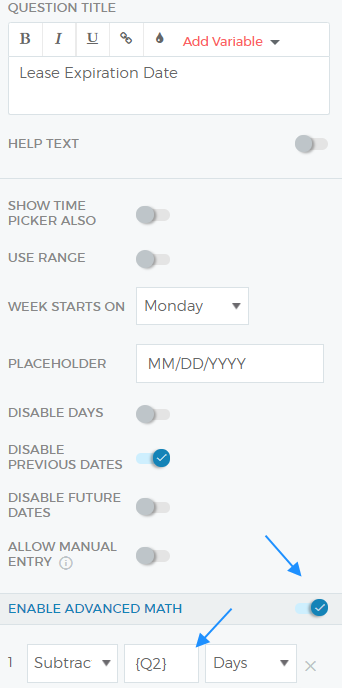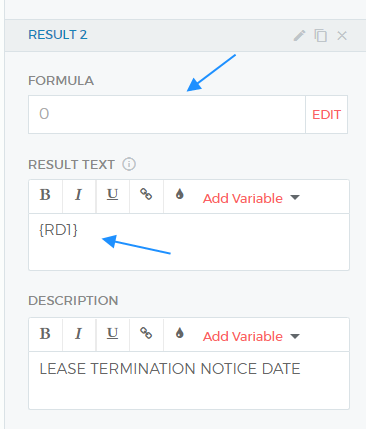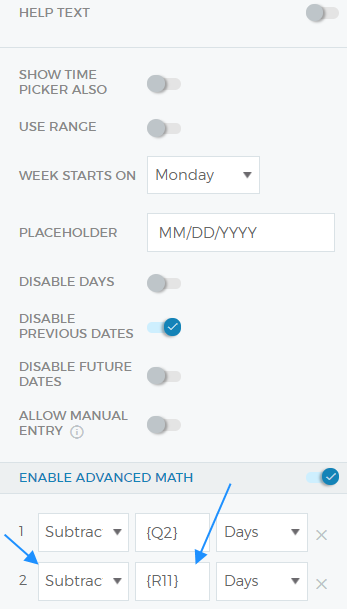# Using 'Advance Math' in Outgrow Formula Builder

You can use the value entered by a user in the Date/Time picker question type as a variable, just need to toggle on the "Enable Advanced Math" setting.

The main advantage of this feature is that it will give you the ability to add or subtract the number of days from the original date picked by a user in order to show a difference.

# To use this functionality in Outgrow, here are the steps that you need to follow:

To use this functionality in Outgrow, here are the steps that you need to follow:

1. The first thing that you need to do is to create a question in your Outgrow content piece with the Date/Time picker as the question type. In this document, we will consider Question 1 as the Date/Time picker question for reference purposes.

2. Now, if you want to subtract the number of days that you have asked in another question from the value selected in Question 1, you need to navigate to Question 1 and then toggle on the "Enable Advanced Maths" setting, and then choose the question whose value needs to be subtracted from Question 1.NOTE - In case you want to use the result calculated in this step and want to display it as a part of the results page text, you can use {RD1} in the result description.3. Another scenario here is that in case you want to add or subtract the number of days calculated using a formula from Q1, then you first need to apply that formula and create a result in the result builder. In this case, let's use this formula as an example ((Q5==0)?0:(Q5+(Q4/500)) and this has been added to calculate R11. Now, in this case, you can use R11 in the "Enable Advanced Maths" section in Q1.NOTE - Now again if you want to use this value in result text, you can use {RD2} as the variable, as this corresponds to the second operation done using Advanced Maths.

Using this mechanism you can use Result and Question variables to subtract/add a given number of days from your original date.

In case you have any questions or concerns, feel free to reach out to us at [email protected] and we will be happy to assist you further.